# [EDX-009] Transfer rate of FTDI's USB3.0 bridge IC FT600

Last Updated: Thursday, 07 September 2017

Here is a demo of measuring transfer rate of FTDI's USB3.0 bridge IC FT600 using an EDX-009.
EDX-009 is an FPGA board which has XILINX's Kintex-7 and FT600をon its credit-card size board.

Transfer rate of FT600 can be calculated as 200MB/s by its 16 bit data width and 100 MHz transfer frequency. An app acts actual trasfer tests and here is a result:

• Transmit  : 190MB/s (average)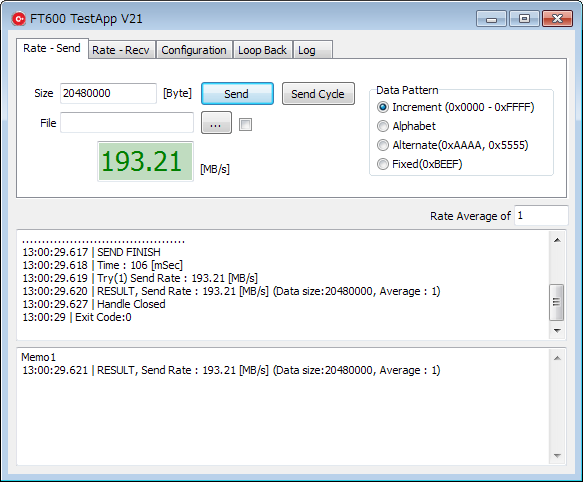Please refer to below for more details.

`Setup`

This test was done with settings below.

• FT600 bus protocol : 245 FIFO at 100MHz
• Data amount (Tx) : 100kB(102400 Byte) to 20,000kB, 200 points in 100kB step
• Data amount (Rx : 100kB(102400 Byte) to 10,000kB, 100 points in 100kB step
• Trials for average : 10
• D3XX device friver version : v1.1.0.0
• Test board : EDX-009

`Transmit Rate`

Periods are timed between after writing data to the FT600 and asserting of complete signal by an API. The FPGA receives data wasting off of them. Here is the graph of result. It gets closer to 190MB.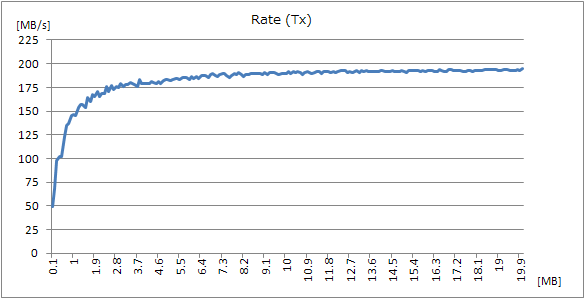Here is some waveforms of FPGA when it received 6000 byte data. Sampling clock is 200MHz.
It shows that the FT600 suspended transmitting after some blocks of data (512/1024/2048 Bytes) by asserting a RXF signal. Period of the suspend is not fixed and around a few to 500 clocks.

Waveform of FPGA receives data (1)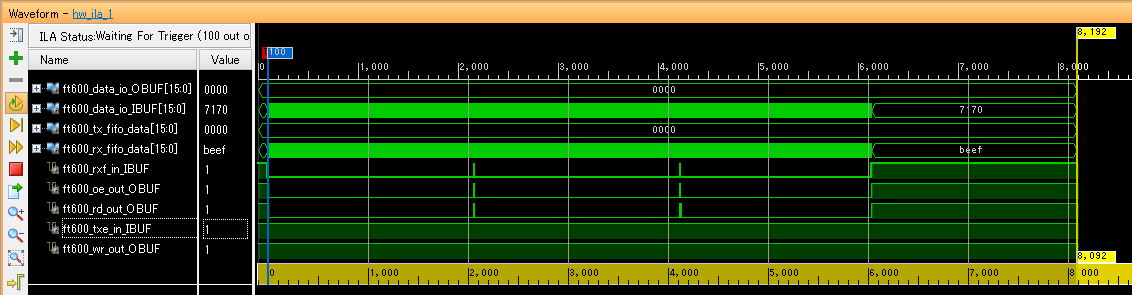Click to enlarge

Waveform of FPGA receives data (2)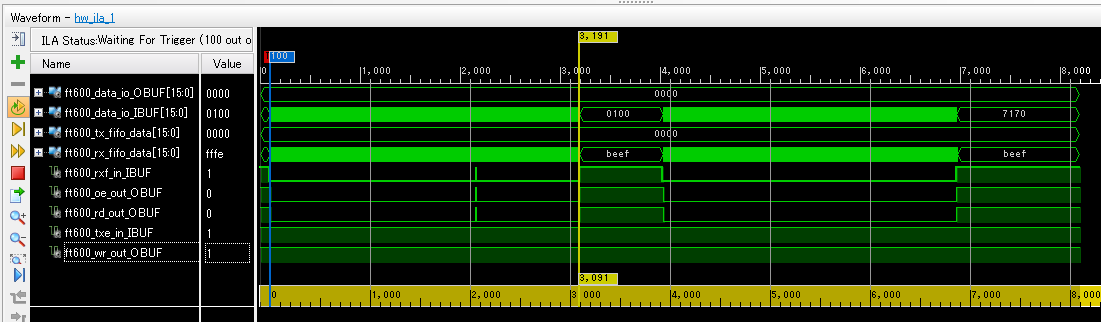Click to enlarge

Waveform of FPGA receives data (3)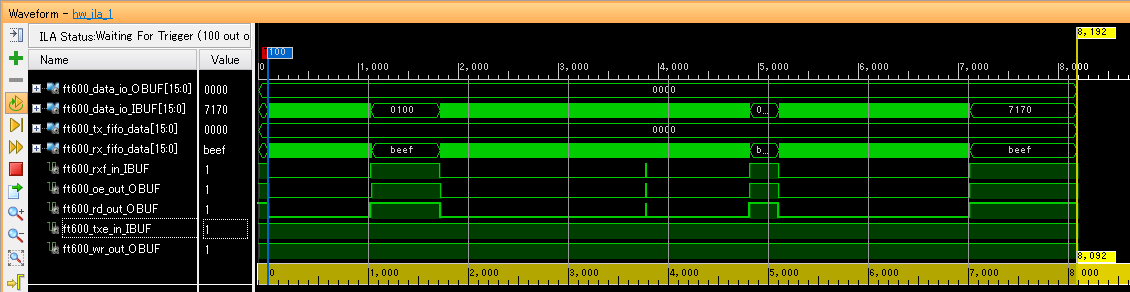Click to enlarge

`Receive Rate`

Periods are timed between requesting to receive data to the FT600 and being informed of completion. Here is the graph of result. It gets closer to 175MB.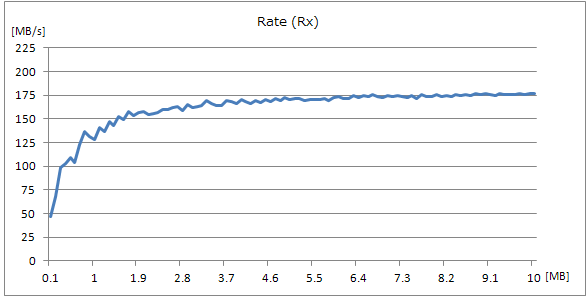The FT600 suspended receiving data after getting 4096 Bytes by asserting an TXE signal. This number is equal to FT600 internal buffer.

Waveform of FPGA transmit data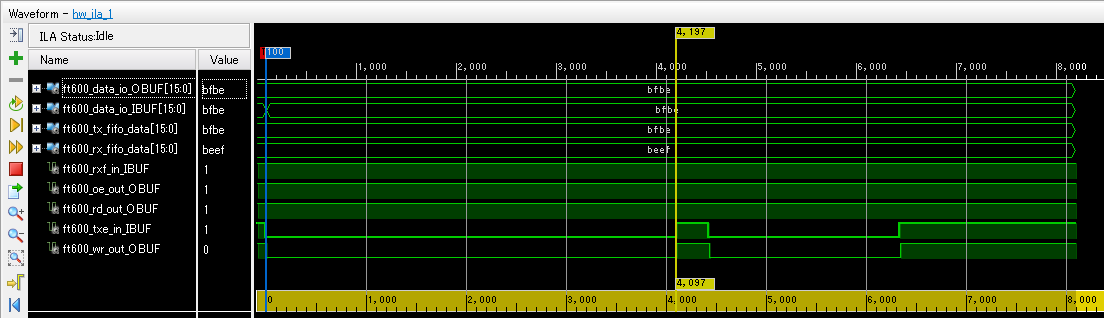Click to enlarge

```Transmit - Application code example
```
```	OVERLAPPED vOverlappedWrite = {0};
vOverlappedWrite.hEvent = CreateEvent(NULL, FALSE, FALSE, NULL);

ftStatus = FT_WritePipe(ftHandle, 0x02, buff, bytesToWrite, &bytesTransferred, &vOverlappedWrite);
if (ftStatus == FT_IO_PENDING)
{
// Poll until all data requested ulBytesToWrite is sent
do
{
// FT_GetOverlappedResult will return FT_IO_INCOMPLETE if not yet finish
ftStatus  =  FT_GetOverlappedResult(ftHandle, &vOverlappedWrite,  &bytesTransferred, FALSE);
if (ftStatus == FT_IO_INCOMPLETE)
{
AttachReport(".");
num_incomp++;
continue;
}
else if(ftStatus != FT_OK)
{
CloseHandle(vOverlappedWrite.hEvent);
return -1;
}
else //if (ftStatus == FT_OK)
{
break;
}
}
while (1);
}
CloseHandle(vOverlappedWrite.hEvent);
```

`Transmit - FPGA state machine example`

The clk is 200MHz. This state machine writes data to the FPGA internal FIFO memory.

```library ieee;
use ieee.std_logic_1164.all;
use ieee.std_logic_unsigned.all;

entity H_FT600_S245_RX_V3 is

port
(
clk    : in std_logic;
reset  : in std_logic;
act    : in std_logic;

rcv_cmp : out std_logic;
rcv_length : out std_logic_vector(15 downto 0);
fifo_data : out std_logic_vector(15 downto 0);
fifo_wrreq : out std_logic;

be     : in std_logic_vector(1 downto 0);
rxf    : in std_logic;
oe     : out std_logic;
rd     : out std_logic;
data   : in std_logic_vector(15 downto 0)
);

end entity;

architecture rtl of H_FT600_S245_RX_V3 is

-- Build an enumerated type for the state machine
type state_type is (idle,s0,s1,s2,s3,s4,s5,s6,t0,t1,e0,e1);
signal state : state_type;
signal rcv_length_reg : std_logic_vector(15 downto 0);
signal rxf_r : std_logic;
signal oe_r  : std_logic;
signal rd_r  : std_logic;

begin

rcv_length <= rcv_length_reg;

process (clk, reset, rxf, act)
begin
if reset = '1' then
state <= idle;
oe <= '1';
rd <= '1';
rcv_cmp <= '0';
rcv_length_reg <= X"0000";
oe_r <= '1';
rd_r <= '1';
fifo_wrreq <= '0';
elsif (rising_edge(clk)) then

rxf_r <= rxf;
oe <= oe_r;
rd <= rd_r;

case state is
when idle =>
rcv_length_reg <= X"0000";
rcv_cmp <= '0';
fifo_data <= X"BEEF";
fifo_wrreq <= '0';
oe_r <= '1';
rd_r <= '1';
if rxf_r = '0' and act = '1' then
state <= s0;
else
state <= idle;
end if;
when s0 =>
state <= s1;
when s1 =>
state <= s2;
when s2 =>
oe_r <= '0';
state <= s3;
when s3 =>
state <= s4;
when s4 =>
rd_r <= '0';
state <= s5;
when s5 =>
state <= s6;
when s6 =>
state <= t0;
---------------------------------------
when t0 =>
if rxf_r = '0' then
fifo_data <= data;
fifo_wrreq <= '1';
oe_r <= '0';
rd_r <= '0';
rcv_length_reg <= rcv_length_reg + 1;
state <= t1;
else
fifo_wrreq <= '0';
oe_r <= '1';
rd_r <= '1';
rcv_length_reg <= rcv_length_reg;
state <= e0;
end if;
when t1 =>
state <= t0;
---------------------------------------
when e0 =>
rcv_cmp <= '1';
state <= e1;
when e1 =>
rcv_cmp <= '0';
state <= idle;
end case;
end if;
end process;
end rtl;
```
```Receive - Application code example
```
```	overlappedRead.hEvent = CreateEventW(NULL, FALSE, FALSE, NULL);

{

if(ftStatus == FT_IO_PENDING){
while(1){
if(ftStatus == FT_IO_INCOMPLETE){
AttachReport(".");
num_incomp++;
continue;
}else if(ftStatus != FT_OK){
break;
}else{ //ftStatus == FT_OK
result = 0;
break;
}
}
}
//----------------------------------------

result = -1;
}
}else{
}
```

`Receive - FPGA state machine example`

The clk is 200MHz. This state machine also reads data from the FPGA internal FIFO memory.

```library ieee;
use ieee.std_logic_1164.all;
use ieee.std_logic_unsigned.all;

entity H_FT600_S245_TX_V6 is

port
(
clk    : in std_logic;
reset  : in std_logic;
act    : in std_logic;

fifo_data : in std_logic_vector(15 downto 0);
fifo_rdreq : out std_logic;
fifo_empty : in std_logic;

be     : inout std_logic_vector(1 downto 0);
txe    : in std_logic;
wr     : out std_logic;
data   : inout std_logic_vector(15 downto 0);
dbg_data   : out std_logic_vector(15 downto 0)
);

end entity;

architecture rtl of H_FT600_S245_TX_V6 is

-- Build an enumerated type for the state machine
type state_type is (idle,s0,s1,s2,t0,t1,e0);
signal state : state_type;
signal fifo_rdreq_w : std_logic;
signal txe_r : std_logic;
signal wr_r : std_logic;

begin

fifo_rdreq <= fifo_rdreq_w and not txe;

process (clk, reset, txe, fifo_data)
begin
if reset = '1' then
state <= idle;
be <= (others=>'Z');
data <= (others=>'Z');
dbg_data <= X"0000";
wr <= '1';
wr_r <= '1';
fifo_rdreq_w <= '0';
elsif (rising_edge(clk)) then

txe_r <= txe;
wr <= wr_r;

case state is
when idle =>
wr_r <= '1';
data <= (others=>'Z');
be <= (others=>'Z');
fifo_rdreq_w <= '0';
if txe_r = '0' and act = '1' then
state <= s0;
else
state <= idle;
end if;
---------------------------------------------
when s0 =>
state <= s1;
when s1 =>
fifo_rdreq_w <= '1';
state <= s2;
when s2 =>
wr_r <= '0';
state <= t0;
--------------------------------------
when t0 =>
if txe_r = '0' then
fifo_rdreq_w <= '1';
wr_r <= '0';
data <= fifo_data;
be <= "11";
dbg_data <= fifo_data;
state <= t1;
else
fifo_rdreq_w <= '0';
wr_r <= '1';
data <= (others=>'Z');
be <= (others=>'Z');
dbg_data <= X"EEEE";
state <= e0;
end if;
when t1 =>
state <= t0;
----------------------------
when e0 =>
state <= idle;
end case;
------------------------------------------
end if;
end process;
end rtl;

```

`Contact us`

We hope you find this demonstration informative.
Please let us know if you notice any mistakes.

Please be forewarned that we do not offer sample design files.

[kw] FT600 EDA-009 EDX-009 USB-106

[ ]

© HuMANDATA LTD. - Intel (Altera) & Xilinx FPGA Boards manufacturer in Japan - 2017 Sitemap